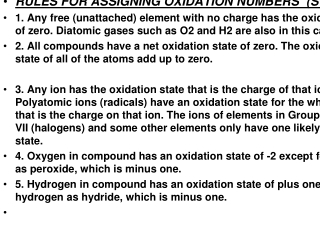Download PresentationRULES FOR ASSIGNING OXIDATION NUMBERS (STATES

# RULES FOR ASSIGNING OXIDATION NUMBERS (STATES

Télécharger la présentation## RULES FOR ASSIGNING OXIDATION NUMBERS (STATES

- - - - - - - - - - - - - - - - - - - - - - - - - - - E N D - - - - - - - - - - - - - - - - - - - - - - - - - - -
##### Presentation Transcript

1. RULES FOR ASSIGNING OXIDATION NUMBERS (STATES • 1. Any free (unattached) element with no charge has the oxidation state of zero. Diatomic gases such as O2 and H2 are also in this category. • 2. All compounds have a net oxidation state of zero. The oxidation state of all of the atoms add up to zero. • 3. Any ion has the oxidation state that is the charge of that ion. Polyatomic ions (radicals) have an oxidation state for the whole ion that is the charge on that ion. The ions of elements in Group I, II, and VII (halogens) and some other elements only have one likely oxidation state. • 4. Oxygen in compound has an oxidation state of -2 except for oxygen as peroxide, which is minus one. • 5. Hydrogen in compound has an oxidation state of plus one, except for hydrogen as hydride, which is minus one.

2. Practice Problem • Assign the oxidation numbers of the atoms in the following compounds. • (a) Al2O3 • (b) XeF4 • (c) K2Cr2O7

3. SOLUTION • (a) The sum of the oxidation numbers in Al2O3 must be zero because the compound is neutral. If we assume that oxygen is present in the -2 oxidation state, the oxidation state of the aluminum must be +3. • Al2O3: 2(+3) + 3(-2) = 0 • (b) Because the oxidation number of the fluorine is -1, the xenon atom must be present in the +4 oxidation state. • XeF4: (+4) + 4(-1) = 0 • (c) Assigning oxidation numbers in this compound is simplified if you recognize that K2Cr2O7 is an ionic compound that contains K+ and Cr2O72- ions. The oxidation state of the potassium is +1 in the K+ ion. Because the oxidation number of oxygen is usually -2, the oxidation state of the chromium in the Cr2O72- ion is +6. • Cr2O7-2: 2(+6) + 7(-2) = -2

4. LOSES ELECTRONS GAINS ELECTRONS REDUCING AGENT OXIDIZING AGENT IS OXIDIZED IS REDUCED

5. GER L LEO

6. HALF REACTIONS A REDOX REACTION MAY BE CONSIDER IN TWO PARTS, ONE REPRESENTING A LOSS OF ELECTRONS (OXIDATION) AND THE OTHER REPRESENTING A GAIN OF ELECTRONS (REDUCTION). EACH REACTION IS KNOWN AS A HALF –REACTION.

7. EXAMPLE Mg(s) + Cl2 → MgCl2 Mg0 → Mg+2 + 2e- (oxidation) / Reducing Agent. Cl2 + 2e- → 2Cl- (reduction) / Oxidizing Agent ____________________________________________ Mg + Cl2 MgCl2

8. Cu + AgNO3 → Cu(NO3)2 + Ag(s) Cu → Cu+2 + 2e- Oxidation/ Reducing Agent Ag+ + e- → Ag Reduction/ Oxidizing Agent 1 [ Cu → Cu+2 + 2e- ] 2 [ Ag+ + e- → Ag ] _____________________________________7 Cu + 2 Ag+ → Cu+2 + Ag (s)

9. Sno + Ag+ → Sn2+ + Ago 1 [ Sn0 → Sn2+ + 2∕e- ] Oxidation 2 [Ag+ + 1∕e- → Ag0 ] Reduction __________________________________________. Sn0 + 2Ag+ → Sn2+ + 2Ag0